Google PageRank algorithm details

What is Google PageRank?

How does it work?

Page Rank is a topic which must be discussed by Search Engine Optimisation (SEO) experts, webmasters, and geeks the world over. At the heart of PageRank is a mathematical formula that seems scary to look at, but is actually fairly simple to understand.

Any good web designer should take the time to fully understand how PageRank really works – if you don’t then your site’s layout could be seriously hurting your Google listings!

PageRank is a link analysis algorithm which assigns a numerical weighting to each element of a hyperlinked set of documents, such as the World Wide Web, with the purpose of “measuring” its relative importance within the set. The algorithm may be applied to any collection of entities with reciprocal quotations and references. The numerical weight that it assigns to any given element E is also called the PageRank of E and denoted by PR(E).

PageRank was developed at Stanford University by Larry Page (hence the name Page-Rank) and Sergey Brin as part of a research project about a new kind of search engine. The project started in 1995 and led to a functional prototype, named Google, in 1998. Shortly after, Page and Brin founded Google Inc., the company behind the Google search engine. While just one of many factors which determine the ranking of Google search results, PageRank continues to provide the basis for all of Google’s web search tools.

 “ PageRank relies on the uniquely democratic nature of the web by using its vast link structure as an indicator of an individual page’s value. In essence, Google interprets a link from page A to page B as a vote, by page A, for page B. But, Google looks at more than the sheer volume of votes, or links a page receives; it also analyzes the page that casts the vote. Votes cast by pages that are themselves “important” weigh more heavily and help to make other pages “important”. ”

In other words, a PageRank results from a “ballot” among all the other pages on the World Wide Web about how important a page is. A hyperlink to a page counts as a vote of support. The PageRank of a page is defined recursively and depends on the number and PageRank metric of all pages that link to it (“incoming links“). A page that is linked to by many pages with high PageRank receives a high rank itself. If there are no links to a web page there is no support for that page.

Numerous academic papers concerning PageRank have been published since Page and Brin’s original paper. In practice, the PageRank concept has proven to be vulnerable to manipulation, and extensive research has been devoted to identifying falsely inflated PageRank and ways to ignore links from documents with falsely inflated PageRank.

Alternatives to the PageRank algorithm are the HITS algorithm proposed by Jon Kleinberg and the IBM CLEVER project.

In early 2005, Google implemented a new value, “nofollow”, for the rel attribute of HTML link and anchor elements, so that website builders and bloggers can make links that Google will not consider for the purposes of PageRank — they are links that no longer constitute a “vote” in the PageRank system. The nofollow relationship was added in an attempt to help combat spamdexing.

As an example, people could create many message-board posts with links to their website to artificially inflate their PageRank. Now, however, the message-board administrator can modify the code to automatically insert “rel=nofollow” to all hyperlinks in posts, thus preventing PageRank from being affected by those particular posts.

The Google Toolbar‘s PageRank feature displays a visited page’s PageRank as a whole number between 0 and 10. Google has not disclosed the precise method for determining a Toolbar PageRank value. Google representatives, such as engineer Matt Cutts, have publicly indicated that the Toolbar PageRank is republished about once every three months, indicating that the Toolbar PageRank values are generally unreliable measurements of actual PageRank value for most periods of year.

and so on…

We can’t know the exact details of the scale because, as we’ll see later, the maximum PR of all pages on the web changes every month when Google does its re-indexing! If we presume the scale is logarithmic (although there is only anecdotal evidence for this at the time of writing) then Google could simply give the highest actual PR page a toolbar PR of 10 and scale the rest appropriately.

The Google Directory PageRank is an 8-unit measurement. These values can be viewed in the Google Directory. Unlike the Google Toolbar which shows the PageRank value by a mouseover of the greenbar, the Google Directory doesn’t show the PageRank values. You can only see the PageRank scale values by looking at the source and wading through the HTML code.

These eight positions are displayed next to each Website in the Google Directory. cleardot.gif is used for a zero value and a combination of two graphics pos.gif and neg.gif are used for the other 7 values. The pixel widths of the seven values are 5/35, 11/29, 16/24, 22/18, 27/13, 32/8 and 38/2 (pos.gif/neg.gif).

Some algorithm details

PageRank is a probability distribution used to represent the likelihood that a person randomly clicking on links will arrive at any particular page. PageRank can be calculated for any-size collection of documents. It is assumed in several research papers that the distribution is evenly divided between all documents in the collection at the beginning of the computational process. The PageRank computations require several passes, called “iterations”, through the collection to adjust approximate PageRank values to more closely reflect the theoretical true value.

A probability is expressed as a numeric value between 0 and 1. A 0.5 probability is commonly expressed as a “50% chance” of something happening. Hence, a PageRank of 0.5 means there is a 50% chance that a person clicking on a random link will be directed to the document with the 0.5 PageRank.

Simplified PageRank algorithm

Suppose a small universe of four web pages: A, B,C and D. The initial approximation of PageRank would be evenly divided between these four documents. Hence, each document would begin with an estimated PageRank of 0.25.

If pages B, C, and D each only link to A, they would each confer 0.25 PageRank to A. All PageRank PR( ) in this simplistic system would thus gather to A because all links would be pointing to A.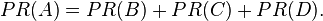$PR(A)= PR(B) + PR(C) + PR(D).\,$

But then suppose page B also has a link to page C, and page D has links to all three pages. The value of the link-votes is divided among all the outbound links on a page. Thus, page B gives a vote worth 0.125 to page A and a vote worth 0.125 to page C. Only one third of D‘s PageRank is counted for A’s PageRank (approximately 0.081).$PR(A)= \frac{PR(B)}{2}+ \frac{PR(C)}{1}+ \frac{PR(D)}{3}.\,$

In other words, the PageRank conferred by an outbound link L( ) is equal to the document’s own PageRank score divided by the normalized number of outbound links (it is assumed that links to specific URLs only count once per document).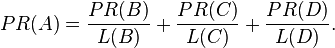$PR(A)= \frac{PR(B)}{L(B)}+ \frac{PR(C)}{L(C)}+ \frac{PR(D)}{L(D)}. \,$

PageRank algorithm including damping factor

The PageRank theory holds that even an imaginary surfer who is randomly clicking on links will eventually stop clicking. The probability, at any step, that the person will continue is a damping factor d. Various studies have tested different damping factors, but it is generally assumed that the damping factor will be set around 0.85.

The damping factor is subtracted from 1 (and in some variations of the algorithm, the result is divided by the number of documents in the collection) and this term is then added to the product of (the damping factor and the sum of the incoming PageRank scores).

That is,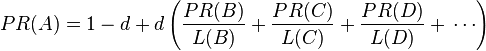$PR(A)= 1 - d + d \left( \frac{PR(B)}{L(B)}+ \frac{PR(C)}{L(C)}+ \frac{PR(D)}{L(D)}+\,\cdots \right)$

or (N = the number of documents in collection)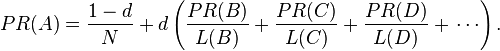$PR(A)= {1 - d \over N} + d \left( \frac{PR(B)}{L(B)}+ \frac{PR(C)}{L(C)}+ \frac{PR(D)}{L(D)}+\,\cdots \right) .$

So any page’s PageRank is derived in large part from the PageRanks of other pages. The damping factor adjusts the derived value downward. The second formula above supports the original statement in Page and Brin’s paper that “the sum of all PageRanks is one”. Unfortunately, however, Page and Brin gave the first formula, which has led to some confusion.

Google recalculates PageRank scores each time it crawls the Web and rebuilds its index. As Google increases the number of documents in its collection, the initial approximation of PageRank decreases for all documents.

The formula uses a model of a random surfer who gets bored after several clicks and switches to a random page. The PageRank value of a page reflects the chance that the random surfer will land on that page by clicking on a link. It can be understood as a Markov chain in which the states are pages, and the transitions are all equally probable and are the links between pages.

If a page has no links to other pages, it becomes a sink and therefore terminates the random surfing process. However, the solution is quite simple. If the random surfer arrives at a sink page, it picks another URL at random and continues surfing again.

When calculating PageRank, pages with no outbound links are assumed to link out to all other pages in the collection. Their PageRank scores are therefore divided evenly among all other pages. In other words, to be fair with pages that are not sinks, these random transitions are added to all nodes in the Web, with a residual probability of usually d = 0.85, estimated from the frequency that an average surfer uses his or her browser’s bookmark feature.

So, the equation is as follows:$PR(p_i) = \frac{1-d}{N} + d \sum_{p_j \in M(p_i)} \frac{PR (p_j)}{L(p_j)}$

where p1,p2,…,pN are the pages under consideration, M(pi) is the set of pages that link to pi, L(pj) is the number of outbound links on page pj, and N is the total number of pages.

The PageRank values are the entries of the dominant eigenvector of the modified adjacency matrix. This makes PageRank a particularly elegant metric: the eigenvector is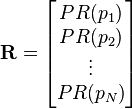$\mathbf{R} = \begin{bmatrix} PR(p_1) \\ PR(p_2) \\ \vdots \\ PR(p_N) \end{bmatrix}$

where R is the solution of the equation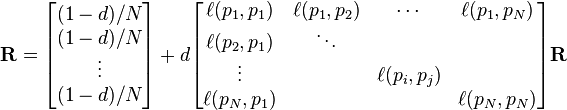$\mathbf{R} = \begin{bmatrix} {(1-d)/ N} \\ {(1-d) / N} \\ \vdots \\ {(1-d) / N} \end{bmatrix} + d \begin{bmatrix} \ell(p_1,p_1) & \ell(p_1,p_2) & \cdots & \ell(p_1,p_N) \\ \ell(p_2,p_1) & \ddots & & \\ \vdots & & \ell(p_i,p_j) & \\ \ell(p_N,p_1) & & & \ell(p_N,p_N) \end{bmatrix} \mathbf{R}$

where the adjacency function$\ell(p_i,p_j)$ is 0 if page pj does not link to pi, and normalised such that, for each j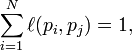$\sum_{i = 1}^N \ell(p_i,p_j) = 1,$

i.e. the elements of each column sum up to 1.

This is a variant of the eigenvector centrality measure used commonly in network analysis.

The values of the PageRank eigenvector are fast to approximate (only a few iterations are needed) and in practice it gives good results.

As a result of Markov theory, it can be shown that the PageRank of a page is the probability of being at that page after lots of clicks. This happens to equal t ? 1 where t is the expectation of the number of clicks (or random jumps) required to get from the page back to itself.

The main disadvantage is that it favors older pages, because a new page, even a very good one, will not have many links unless it is part of an existing site (a site being a densely connected set of pages). The Google Directory (itself a derivative of the Open Directory Project) is an exception in which PageRank is not used to determine search results rankings.

Several strategies have been proposed to accelerate the computation of PageRank.

Various strategies to manipulate PageRank have been employed in concerted efforts to improve search results rankings and monetize advertising links. These strategies have severely impacted the reliability of the PageRank concept, which seeks to determine which documents are actually highly valued by the Web community.

Google is known to actively penalize link farms and other schemes designed to artificially inflate PageRank. How Google identifies link farms and other PageRank manipulation tools are among Google’s trade secrets.

False or spoofed PageRank

Google’s home page is often considered to be automatically rated a 10/10 by the Google Toolbar‘s PageRank feature, but its PageRank has at times shown a surprising result of only 8/10.

Other uses of PageRank

A version of PageRank has recently been proposed as a replacement for the traditional ISI impact factor. Instead of merely counting citations of a journal, the “quality” of a citation is determined in a PageRank fashion.

A Web crawler may use PageRank as one of a number of importance metrics it uses to determine which URL to visit next during a crawl of the web. One of the early working papers which was used in the creation of Google is Efficient crawling through URL ordering, which discusses the use of a number of different importance metrics to determine how deeply, and how much of a site Google will crawl. PageRank is presented as one of a number of these importance metrics, though there are others listed such as the number of inbound and outbound links for a URL, and the distance from the root directory on

Finally

PageRank is, in fact, very simple (apart from one scary looking formula). But when a simple calculation is applied hundreds (or billions) of times over the results can seem complicated.

PageRank is also only part of the story about what results get displayed high up in a Google listing. For example there’s some evidence to suggest that Google is paying a lot of attention these days to the text in a link’s anchor when deciding the relevance of a target page – perhaps more so than the page’s PR.

PageRank is still part of the listings story though, so it’s worth your while as a good designer to make sure you understand it correctly. A really great website is designed with Page Rank and other factors in mind from the start in order to make it truly Competitive in the search engines.

1.Check Pagerank Says:
2.Mix Music Online Says: